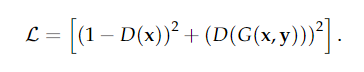# Which function a generator should maximize during GAN training?

I have a GAN loss function for image style transfer. Let ‘x’ here be the content image that needs to be stylized and ‘y’ be the style image.Now, the discriminator D() is trying to minimize this function while at the same time the generator G() is trying to maximize it. So while implementing the code for this loss function, will the discriminator function take both the terms into consideration and try to minimize the sum? And the generator function will be concerned with only the second part of the equation that involves the generated image and try to maximize it?
Did I get it right?